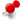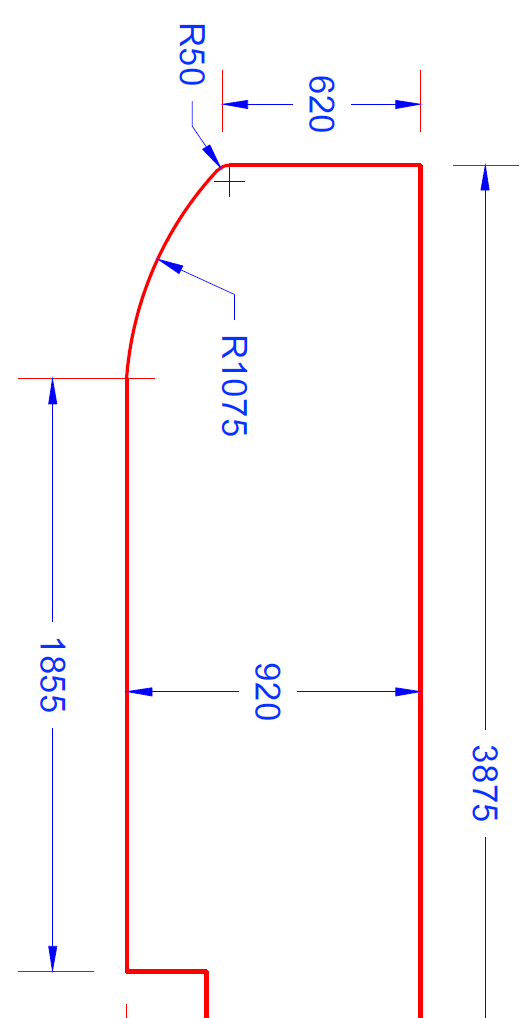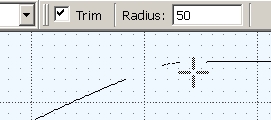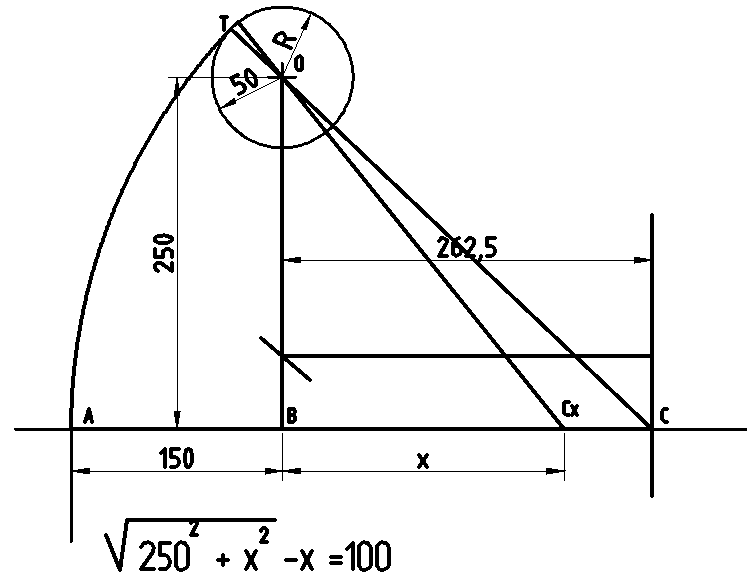# Tangent Curve between existing circle and lineClassicListThreaded3 messagesOpen this post in threaded view
|

## Tangent Curve between existing circle and lineI Need some help constructing the below shape... i have no issue creating the 2 tangent curves from the end of each of the straight lines However, how do you then get the 2 curves to them meet at their tangent also?
 Modify - Fillet  (with Trim, and radius set at the Tool Options bar).In reply to this post by Juniorscone the solution need some calculations See the imageThe center of arc is on the line AB C, O and T are aligned AB and OB are known The radius of arc is the sum of CO and R CO is calculated by Pytagore theorem Finaly we obtain the équation at the bottom of the picture Solving this équation is easy placing the square root in a side and the rest on the other by sqaring all. We obtain x = 262.5 The radius of the arc is 150 + 262.5 Point C is the center of the arc.